# Discovering Pick’s Formula

Finding the area of polygons is pretty easy if the shapes are familiar to you like rectangles, triangles, circles, etc. Those shapes have nice formulas we can learn that will give us the area of the figure without much hassle.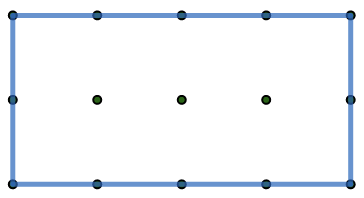But what about unusual shapes like this?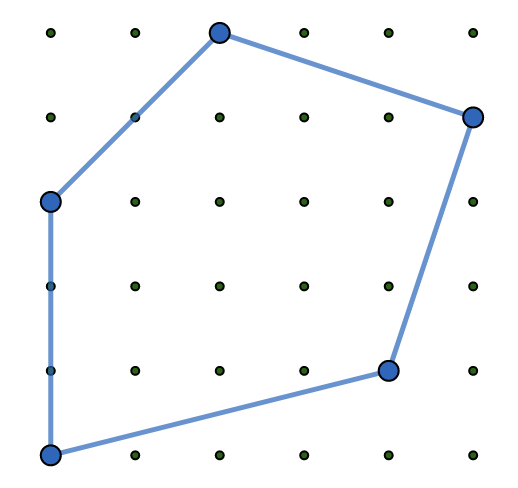Sure we could find the area of this polygon by cutting it up into smaller, more familiar shapes and then adding up all the areas. Like so…Hey look at that…the area of that polygon is 17 square units!

But wouldn’t it be nice if there was a formula that would give us the area with a minimal amount of work? In fact, there is such a formula…it is called Pick’s Formula. I’m sure a quick jaunt to Wikipedia would tell us who Pick is, but let’s just jump into discovering the formula!

When you look at a figure that is on a grid, it seems that the grid dots are either ON the figure or IN the figure.

Dots are ON the figure if the side lengths of the polygon go through the line.

Dots are IN the figure if they are inside the polygon.Let’s find a relationship between the grid dots and the area of any polygon.

We will start small and work our way up to larger figures. All the while we will collect our data in a table to find patterns. Hopefully we will discover the relationship.

In this 1×1 figure, there are 4 dots ON the figure and 0 dots in the figure. The area is 1 square unit.

 Dimensions Dots ON Dots IN Area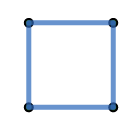1 x 1 4 0 1

Now lets add a few more carefully sequenced figures to the table.

 Dimensions Dots ON Dots IN Area1 x 1 4 0 1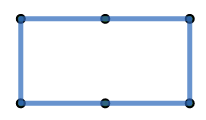1 x 2 6 0 2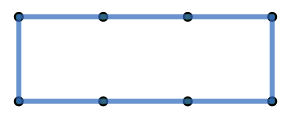1 x 3 8 0 3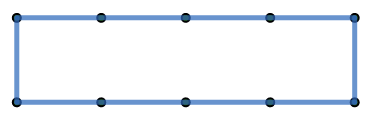1 x 4 10 0 4

What patterns do we notice? Perhaps you see the dots ON goes up by 2 each time while the area goes up by 1 each time.

Can you see a relationship between the dots ON and the area? For each figure the area is one less than half of the dots ON. The formula so far seems to be

$$\frac{dotsON}{2} – 1 = Area$$

Let’s try a new collection of polygons that all have a height of 2 units, beginning with 2×1.

 Dimensions Dots ON Dots IN Area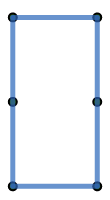2 x 1 6 0 2

Well this isn’t all that surprising since we already had the data collected previously with the 1×2. But let’s continue with other rectangles that are 2 units high.

 Dimensions Dots ON Dots IN Area2 x 1 6 0 2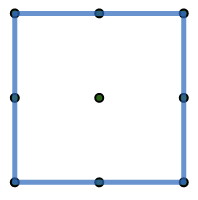2 x 2 8 1 4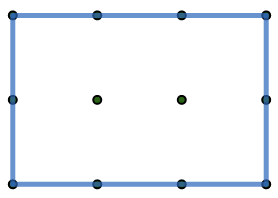2 x 3 10 2 62 x 4 12 3 8

How do we need to revise our original formula to work with this new collection of data?

Let’s look at 2×2.

 Dimensions Dots ON Dots IN Area2 x 2 8 1 4

Our original formula is $$\frac{dotsON}{2} – 1 = Area$$

Substituting our new information for the 2 by 2 gives us $$\frac{8}{2} – 1 = 3$$, but we wanted the answer to be 4, which is off by 1. Hey…there also happens to be 1 dot IN!

 Dimensions Dots ON Dots IN Area2 x 3 10 2 6

Let’s try 2 by3. Substituting the dots ON and In for 2 by 3 gives us

$$\frac{10}{2} – 1 = 4$$

but we wanted the answer to be 6, which is off by 2. Two is the number of dots IN!

Each time we use the formula $$\frac{dotsON}{2} – 1 = Area$$  we need to also add in the dots IN to get the correct area.

Our formula, therefore, seems to be

$$\frac{dotsON}{2} – 1 + {dotsIN}= Area$$

Let’s confirm our suspicion with the 2×4 rectangle.

 Dimensions Dots ON Dots IN Area2 x 4 12 3 8

Here’s the formula so far $$\frac{dotsON}{2} – 1 + {dotsIN}= Area$$.

Now substitute in the data $$\frac{12}{2} – 1 + {3}= 8$$

Hey it works!!!

Let’s try our new formula with a 3×5 rectangle to confirm that our formula works.

 Dimensions Dots ON Dots IN Area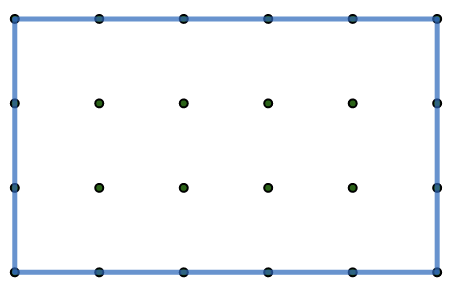3 x 5 16 8 15

$$\frac{dotsON}{2} – 1 + {dotsIN} = \frac{16}{2} – 1 + {8} = 15$$

Now let’s go back to that original crazy figure with an area of 17 square units and see if our formula still works.

 Dots ON Dots IN Area8 14 ??

$$\frac{dotsON}{2} – 1 + {dotsIN} = \frac{8}{2} – 1 + {14} = 17$$

Woo hoo! The formula works even with unusual shapes! Let’s celebrate by putting it in an important looking table…

 Pick’s Formula $$\frac{dots ON}{2} – 1 + {dots IN} = {Area}$$

For further celebration, use your new formula to find the area of this figure…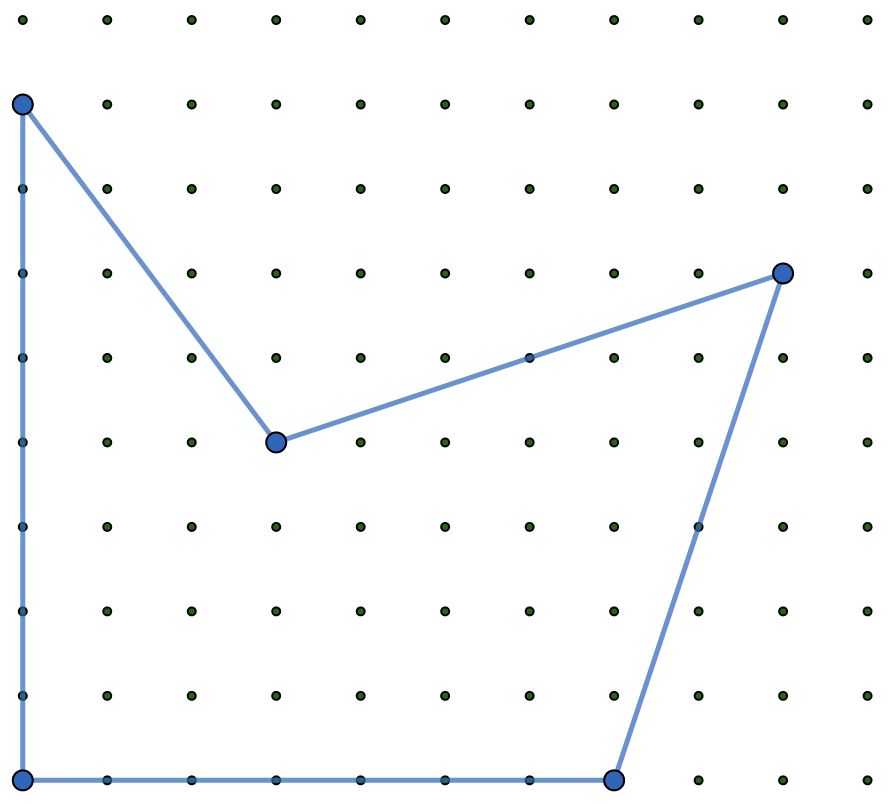You can even use Pick’s Formula to estimate areas of curvy shapes on the grid but I’ll leave that for another chapter.

.

.

.

1.Traci Breedlove says: